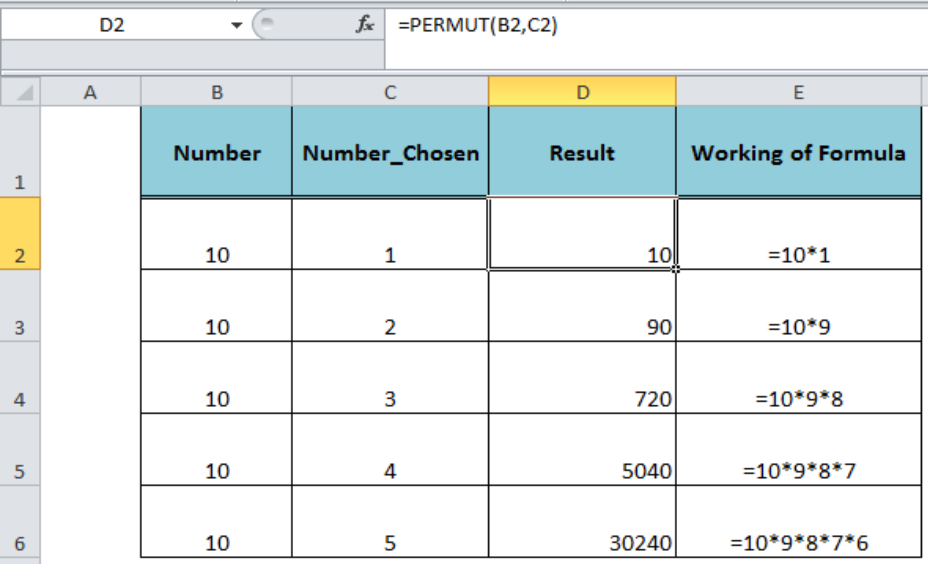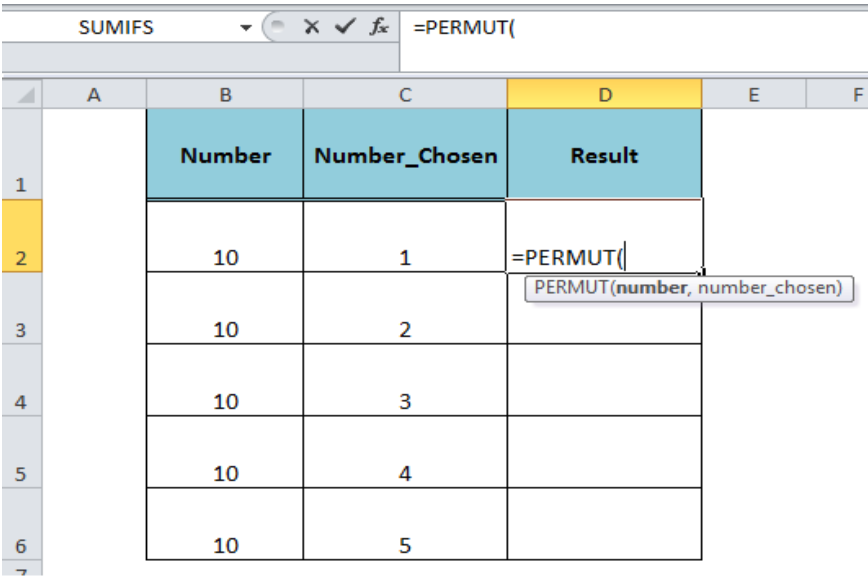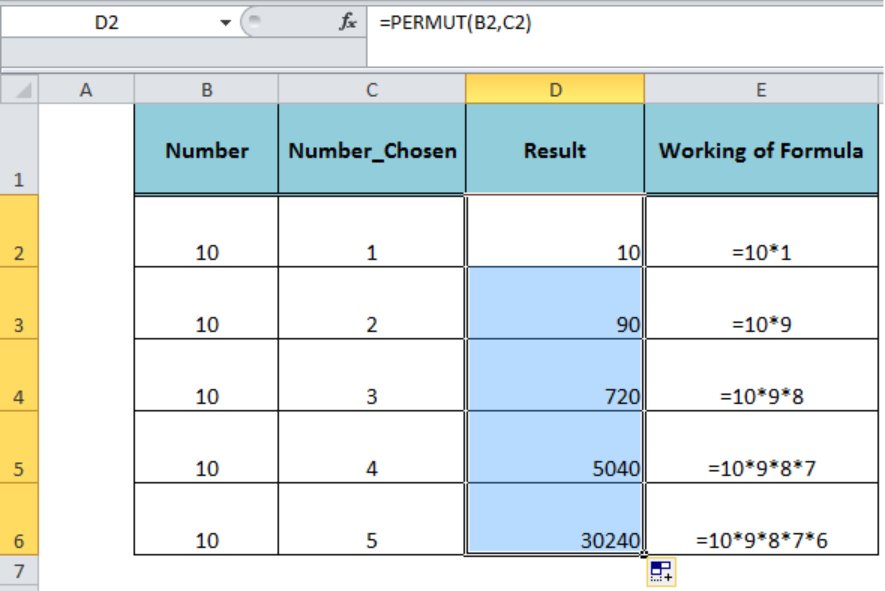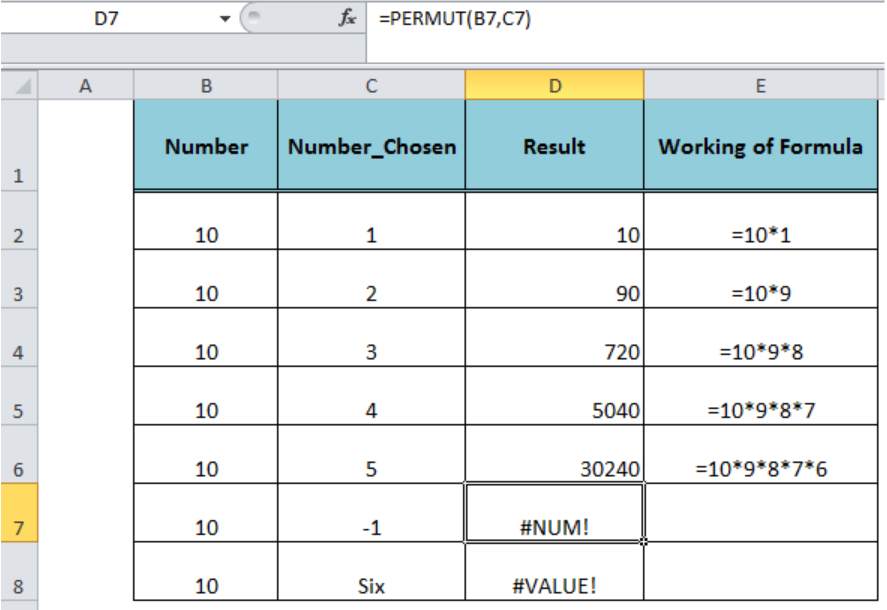Get instant live expert help with Excel or Google Sheets“My Excelchat expert helped me in less than 20 minutes, saving me what would have been 5 hours of work!”

#### Post your problem and you’ll get Expert help in seconds.

Your message must be at least 40 characters
Our professional Expert are available now. Your privacy is guaranteed.

# Excel PERMUT Function

The Excel PERMUT function calculates the number of permutations of a specified number from a given set of number. The permutation is basically the calculation of the number of ways the arrangement of all the items of a set can be made where the order of arrangement is very important.Figure 1. How to Use the PERMUT Function

## Syntax

The syntax for the Excel PERMUT function is;

`=PERMUT(number, number_chosen)`

Where,

• Number, the argument is the total number of items or events
• Number_chosen, the argument is the number of items in each permutation or arrangement.Figure 2. The Syntax for the Excel PERMUT Function

## Example

Let’s say a company wants to start its operation across the country. The company aims to initiate its operation sequentially for a specified number (Number_Chosen) of locations out of total 10 locations. Because the company the operations will be started sequentially, so the order of arrangements is important and we need to calculate the permutation using the PERMUT function. We will calculate the permutation in column D as per the following formula and copy down to other cells:

`=PERMUT(B2,C2)`Figure 3. Applying the PERMUT Function

## Notes

• The permutation is closely related to the combination, but in combination, the order is not important.
• If the value of Number or Number_Chosen argument is not an integer, then it is truncated.
• The function returns #NUM! error, If any of the arguments, Number or Number_Chosen, is less than zero “<0”.
• It returns #VALUE! error, if any of the arguments is not a numeric value.Figure 4. Error Values of the PERMUT Function

## Instant Connection to an Expert through our Excelchat Service

Most of the time, the problem you will need to solve will be more complex than a simple application of a formula or function. If you want to save hours of research and frustration, try our live Excelchat service! Our Excel Experts are available 24/7 to answer any Excel question you may have. We guarantee a connection within 30 seconds and a customized solution within 20 minutes.